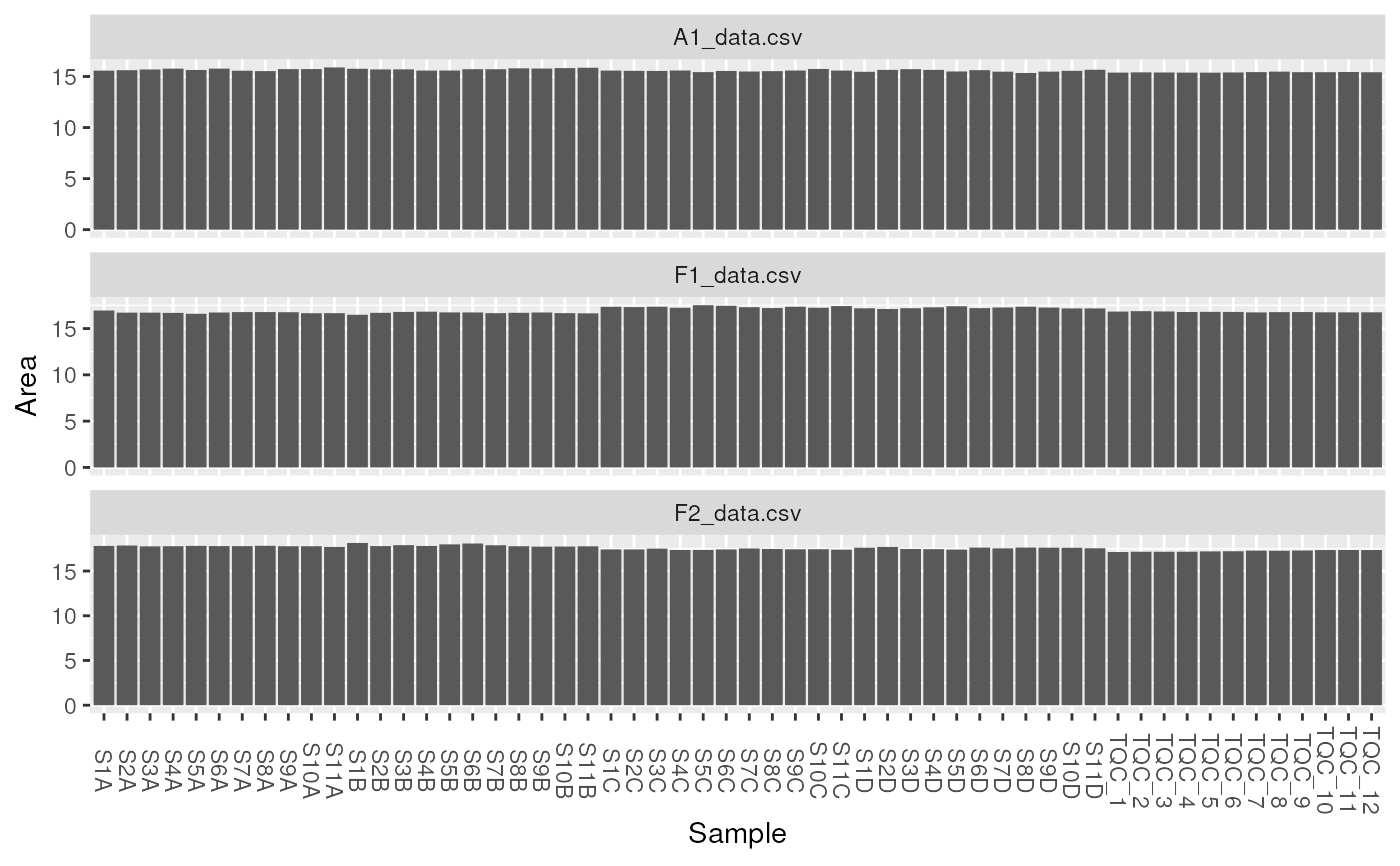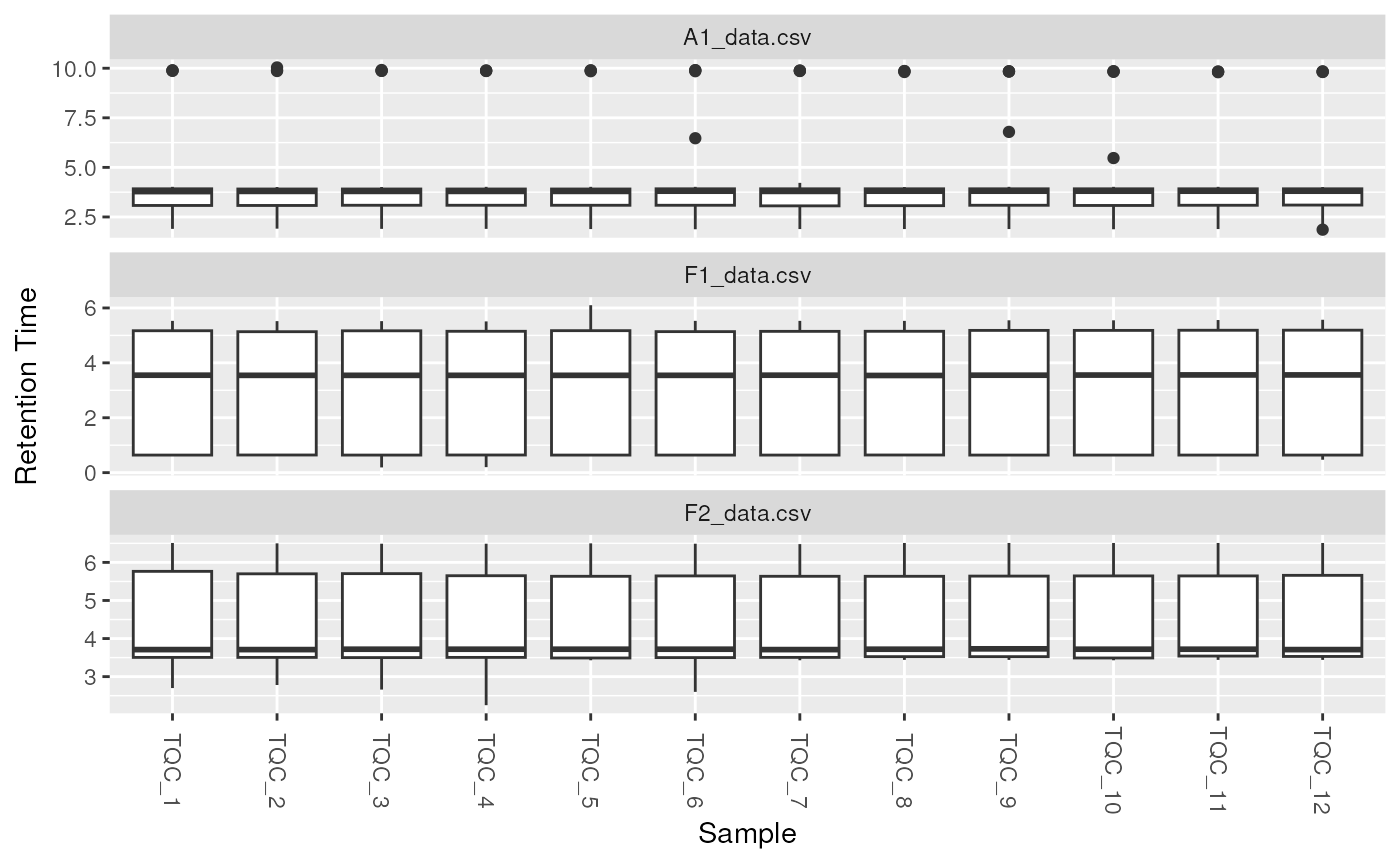lipidr supports two types of plots for sample quality checking.

tic plots a bar chart for total sample intensity.

boxplot plots a boxplot chart to examine the distribution of values per sample.

plot_samples(
data,
type = c("tic", "boxplot"),
measure = "Area",
log = TRUE,
color = NULL
)

## Arguments

data

LipidomicsExperiment object.

type

plot type, either tic or boxplot. Default is tic.

measure

Which measure to use as intensity, usually Area, Area Normalized or Height. Default is Area

log

Whether values should be log2 transformed. Default is TRUE

color

The column name of a sample annotation to be used as color

A ggplot object.

## Examples

data(data_normalized)

plot_samples(data_normalized, type = "tic", "Area", log = TRUE)plot_samples(data_normalized, type = "tic", "Background", log = FALSE)
#> Warning: Removed 1 rows containing non-finite values (stat_summary).plot_samples(
data_normalized[, data_normalized\$group == "QC"],
type = "boxplot",
measure = "Retention Time", log = FALSE
)
#> Warning: Removed 1 rows containing non-finite values (stat_boxplot).